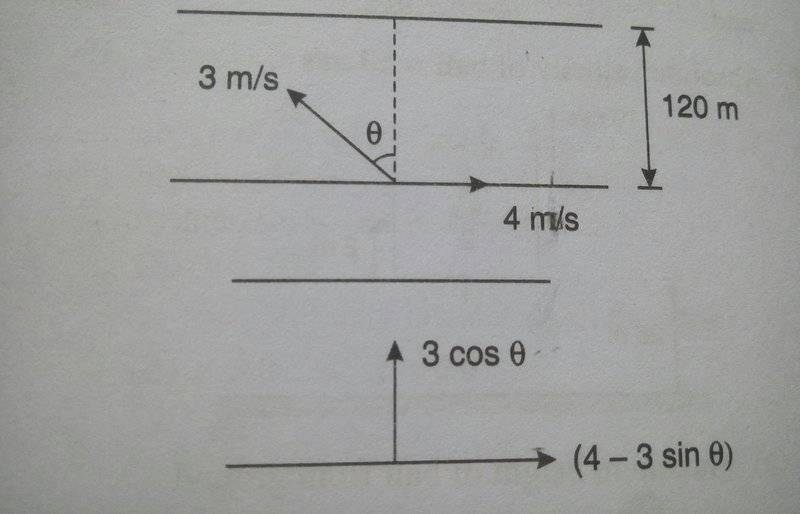# River-boat problem (Relative velocity)

## Homework Statement

A man wishes to cross a river of width 120 m by a motorboat. His rowing speed in still water (or relative to water) is 3 m/s and his maximum walking speed is 1 m/s. The river flows with a velocity of 4 m/s.
(a) Find the path which he should take to reach the point directly opposite to his starting point in the shortest time.
(b) Find the time required to reach the destination.2. Homework Equations

Let ω be the width of the river and x be the drifting of the boat.
Let v denote the velocity vectors and v denote their respective magnitudes.
vr = absolute velocity of river
vbr = velocity of boatman relative to the river or velocity of boatman in still water
vb = absolute velocity of boatman
Hence, vbr = vb - vr
vb = vbr + vr
Time taken to cross the river t = ω/vbr-y = ω/vbrcos θ
Drift x = vb-xt = (vr - vbrsin θ)ω/vbrcos θ

## The Attempt at a Solution

Now, in order to reach the point directly opposite to his starting point, resultant velocity vb must be perpendicular to the river current.
So, for this the drift x must be 0.
x = 0 gives vr = vbrsin θ
Subsituting, sin θ = 4/3, which is meaningless.
I can't proceed without θ. Also, I don't get why they've given his maximum walking speed.
Am I missing anything?

#### Attachments

Last edited:

Orodruin
Staff Emeritus
Homework Helper
Gold Member
2021 Award
You are missing that a 3 m/s velocity cannot have a 4 m/s component so regardless of how he rows he will have to walk back on the other side. This is why you are given the walking speed. The angle ##\theta## is a parameter and you will obtain different results for different angles. You are asked to find the shortest time possible so you need to minimise the time as a fuction of the angle.

So, t = 120/3cos θ = 40/cos θ
x = (4 - 3sin θ)40 sec θ = 160sec θ - 120tan θ
But I can't just differentiate t = 40sec θ. That wouldn't render anything...

Orodruin
Staff Emeritus
Homework Helper
Gold Member
2021 Award
Please define your variables properly. If not you are just assuming we know what you are doing, but we will only be guessing. In particular, what is your definition of the time t and what is its relation to your sought time?

Please define your variables properly. If not you are just assuming we know what you are doing, but we will only be guessing. In particular, what is your definition of the time t and what is its relation to your sought time?
Of course, it's the time taken to cross the river. We must find the shortest time possible, which, as you said, must be minimised as a function of the angle.
But I still don't get how to apply the walking speed and what to do with the drift equation.

I have the drift of the boat as a function of the angle.

jbriggs444
Homework Helper
Of course, it's the time taken to cross the river. We must find the shortest time possible, which, as you said, must be minimised as a function of the angle.
We are not asked to minimize the time taken to cross the river. We are asked to minimize the time taken to reach a point opposite the starting point.

Since the boat is guaranteed to drift downstream as it is rowed across the river, the man must either walk the boat upstream before launching or after landing on the opposite bank. How far he has to walk it will depend on the angle at which he rows.

How far he has to walk it will depend on the angle at which he rows.
So we take x to be the drift of the man and then find the time (of course as a function of the angle)?
That means time of walking t| = x/vwalking
∴ t| = (160sec θ - 120tan θ)/1
total time = t + t| = 200sec θ - 120tan θ
And, then you minimize it? Does that sound right?

jbriggs444
Homework Helper
So we take x to be the drift of the man and then find the time (of course as a function of the angle)?
That means time of walking t| = x/vwalking
∴ t| = (160sec θ - 120tan θ)/1
total time = t + t| = 200sec θ - 120tan θ
And, then you minimize it? Does that sound right?
WIthout having carefully gone over the arithmetic, yes, that sounds right.

It has the right qualitative feel. It has the 200 sec θ which embodies the elapsed time crossing the river plus the extra time needed to compensate for downstream drift. It has the -120 tan θ which embodies the limited advantage one can get by aiming upstream to reduce the drift velocity.

WIthout having carefully gone over the arithmetic, yes, that sounds right.

It has the right qualitative feel. It has the 200 sec θ which embodies the elapsed time crossing the river plus the extra time needed to compensate for downstream drift. It has the -120 tan θ which embodies the limited advantage one can get by aiming upstream to reduce the drift velocity.
That would yield θ = sin-1 (3/5) and t = 160 s.
Thanks